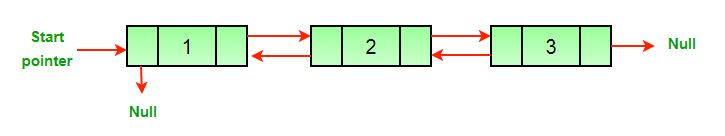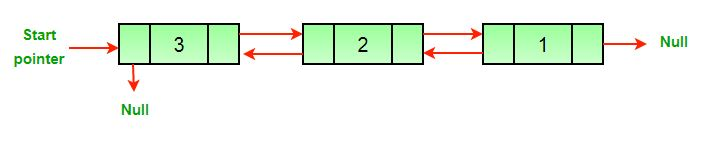# Java Program For Reversing A Doubly Linked List

• Last Updated : 14 Dec, 2021

See below diagrams for example.

`(a) Original Doubly Linked List  ``(b) Reversed Doubly Linked List  `Here is a simple method for reversing a Doubly Linked List. All we need to do is swap prev and next pointers for all nodes, change prev of the head (or start) and change the head pointer in the end.

## Java

 `// Java program to reverse a doubly ``// linked list``class` `LinkedList ``{``    ``static` `Node head;``    ``static` `class` `Node ``    ``{``        ``int` `data;``        ``Node next, prev;``        ``Node(``int` `d)``        ``{``            ``data = d;``            ``next = prev = ``null``;``        ``}``    ``}`` ` `    ``/* Function to reverse a Doubly ``       ``Linked List */``    ``void` `reverse()``    ``{``        ``Node temp = ``null``;``        ``Node current = head;`` ` `        ``/* Swap next and prev for all nodes ``           ``of doubly linked list */``        ``while` `(current != ``null``) ``        ``{``            ``temp = current.prev;``            ``current.prev = current.next;``            ``current.next = temp;``            ``current = current.prev;``        ``}`` ` `        ``/* Before changing head, check for the ``           ``cases like empty list and list with ``           ``only one node */``        ``if` `(temp != ``null``) ``        ``{``            ``head = temp.prev;``        ``}``    ``}`` ` `    ``// UTILITY FUNCTIONS ``    ``/* Function to insert a node at the ``       ``beginning of the Doubly Linked List */``    ``void` `push(``int` `new_data)``    ``{``        ``// Allocate node ``        ``Node new_node = ``new` `Node(new_data);`` ` `        ``/* Since we are adding at the beginning,``           ``prev is always NULL */``        ``new_node.prev = ``null``;`` ` `        ``// Link the old list off the new node ``        ``new_node.next = head;`` ` `        ``/* Change prev of head node to ``           ``new node */``        ``if` `(head != ``null``) {``            ``head.prev = new_node;``        ``}`` ` `        ``/* Move the head to point to the ``           ``new node */``        ``head = new_node;``    ``}`` ` `    ``/* Function to print nodes in a given ``       ``doubly linked list. This function is ``       ``same as printList() of singly linked``       ``list */``    ``void` `printList(Node node)``    ``{``        ``while` `(node != ``null``) ``        ``{``            ``System.out.print(node.data + ``" "``);``            ``node = node.next;``        ``}``    ``}`` ` `    ``public` `static` `void` `main(String[] args)``    ``{``        ``LinkedList list = ``new` `LinkedList();`` ` `        ``/* Let us create a sorted linked list ``           ``to test the functions Created linked ``           ``list will be 10->8->4->2 */``        ``list.push(``2``);``        ``list.push(``4``);``        ``list.push(``8``);``        ``list.push(``10``);`` ` `        ``System.out.println(``        ``"Original linked list "``);``        ``list.printList(head);`` ` `        ``list.reverse();``        ``System.out.println(``""``);``        ``System.out.println(``        ``"The reversed Linked List is "``);``        ``list.printList(head);``    ``}``}``// This code is contributed by Mayank Jaiswal`

Output:

```Original linked list
10 8 4 2
2 4 8 10```

Time Complexity: O(N), where N denotes the number of nodes in the doubly linked list.
Auxiliary Space: O(1)
We can also swap data instead of pointers to reverse the Doubly Linked List. Method used for reversing array can be used to swap data. Swapping data can be costly compared to pointers if the size of the data item(s) is more.
Please write comments if you find any of the above codes/algorithms incorrect, or find better ways to solve the same problem.

Method 2:

The same question can also be done by using Stacks.

Steps:

1. Keep pushing the node’s data in the stack. -> O(n)
2. The keep popping the elements out and updating the Doubly Linked List

## Java

 `// Java program to reverse a doubly ``// linked list``import` `java.util.*;``class` `LinkedList ``{``    ``static` `Node head;`` ` `    ``static` `class` `Node ``    ``{``        ``int` `data;``        ``Node next, prev;`` ` `        ``Node(``int` `d)``        ``{``            ``data = d;``            ``next = prev = ``null``;``        ``}``    ``}`` ` `    ``/* Function to reverse a Doubly Linked ``       ``List using Stacks */``    ``void` `reverse()``    ``{``        ``Stack stack = ``new` `Stack<>();``        ``Node temp = head;``        ``while` `(temp != ``null``) ``        ``{``            ``stack.push(temp.data);``            ``temp = temp.next;``        ``}``        ``// Added all the elements sequence ``        ``// wise in the stack``        ``temp = head;``        ``while` `(temp != ``null``) ``        ``{``            ``temp.data = stack.pop();``            ``temp = temp.next;``        ``}`` ` `        ``// Popped all the elements and the ``        ``// added in the linked list,``        ``// which are in the reversed order.``    ``}`` ` `    ``// UTILITY FUNCTIONS ``    ``/* Function to insert a node at the ``       ``beginning of the Doubly Linked List */``    ``void` `push(``int` `new_data)``    ``{``        ``// Allocate node ``        ``Node new_node = ``new` `Node(new_data);`` ` `        ``/* Since we are adding at the beginning,``           ``prev is always NULL */``        ``new_node.prev = ``null``;`` ` `        ``/* Link the old list off the new node */``        ``new_node.next = head;`` ` `        ``/* Change prev of head node to ``           ``new node */``        ``if` `(head != ``null``) ``        ``{``            ``head.prev = new_node;``        ``}`` ` `        ``/* move the head to point to the new node */``        ``head = new_node;``    ``}`` ` `    ``/* Function to print nodes in a given doubly linked list``     ``This function is same as printList() of singly linked``     ``list */``    ``void` `printList(Node node)``    ``{``        ``while` `(node != ``null``) {``            ``System.out.print(node.data + ``" "``);``            ``node = node.next;``        ``}``    ``}`` ` `    ``// Driver Code``    ``public` `static` `void` `main(String[] args)``    ``{``        ``LinkedList list = ``new` `LinkedList();`` ` `        ``/* Let us create a sorted linked list to test the``         ``functions Created linked list will be 10->8->4->2``       ``*/``        ``list.push(``2``);``        ``list.push(``4``);``        ``list.push(``8``);``        ``list.push(``10``);`` ` `        ``System.out.println(``        ``"Original linked list "``);``        ``list.printList(head);`` ` `        ``list.reverse();``        ``System.out.println(``""``);``        ``System.out.println(``        ``"The reversed Linked List is "``);``        ``list.printList(head);``    ``}``}``// This code is contributed by Rashita Mehta`

Output:

```Original linked list
10 8 4 2